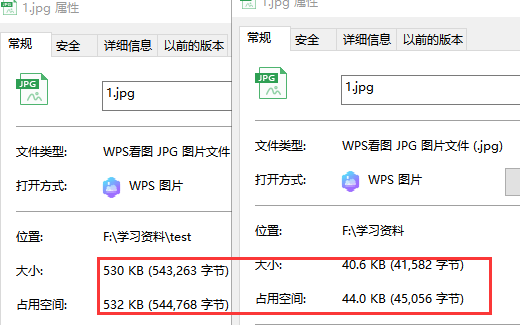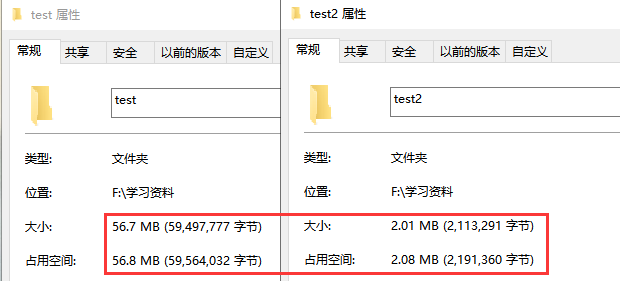# 7行代码实现图片等比例压缩（python）

#### 先把标题的功能做出来（我不是标题党的呀！）

from PIL import Image

file = '1.jpg'
img = Image.open(file)
w,h = img.size
w,h = round(w * 0.2),round(h * 0.2)		// 去掉浮点，防报错

img = img.resize((w,h), Image.ANTIALIAS)
img.save('1.jpg', optimize=True, quality=85)	// 质量为85效果最好#### 再来试试指定宽度按比例进行批量压缩

• 按指定宽度自适应高度
• 批量压缩

newWidth = 600
newHeight = round(newWidth / w * h)


import os
from PIL import Image

dirPath = 'test/'
allFile = os.listdir(dirPath)
for i in allFile:
file = dirPath + i
img = Image.open(file)
w,h = img.size

newWidth = 600
newHeight = round(newWidth / w * h)

img = img.resize((newWidth,newHeight), Image.ANTIALIAS)
img.save('test2/%s' % i, optimize=True, quality=85)#### 最后©️2019 CSDN 皮肤主题: 精致技术 设计师: CSDN官方博客